## What is Hall Effect in Semiconductor?:

When a specimen (metal or semiconductor) is placed in a transverse magnetic field and a direct current is passed through it, then an electric field is induced across its edges in the perpendicular direction of current as well as magnetic field. This phenomenon is called the Hall effect in Semiconductor.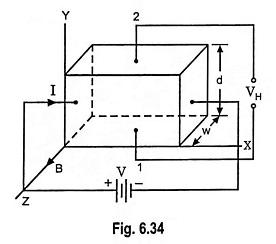A semiconductor bar carrying a current I in the positive X-direction and placed in a magnetic field B acting in the positive Z direction is illustrated in Fig. 6.34. Now a force is exerted on the charge carriers (electrons or holes) in the negative Y direction. If the semiconductor is of N-type, so that the current is carried by electrons, these electrons will be forced downward toward side 1 and side 1 becomes negatively charged with re­spect to side 2. Thus a potential difference VH, called the Hall voltage, is developed between surfaces 1 and 2. The polarity of Hall voltage enables us to determine whether the semiconductor specimen is N-type or P-type. In case of P-type semiconductor side 1 will become positively charged with respect to side 2.

In the equilibrium state the electric field intensity E due to Hall Effect in Semiconductor must exert a force on the charge carriers which just balances the magnetic force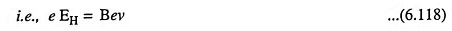where e is the magnitude of charge on electron or hole and v is the drift velocity.

Now electric field,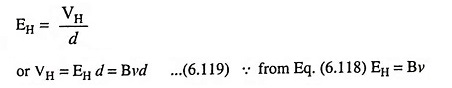where d is the distance between surfaces 1 and 2.

The current density is given as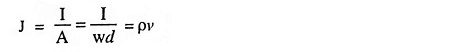where ρ is the charge density and w is the width of specimen.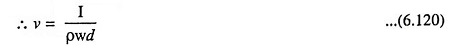From Eqs. (6.119) and (6.120), we have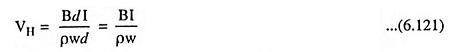Thus from the above equation charge density ρ can be determined if the quantities B, I, VH and w are measured.

Hall field per unit current density per unit magnetic field is called the Hall coefficient.

So Hall coefficient,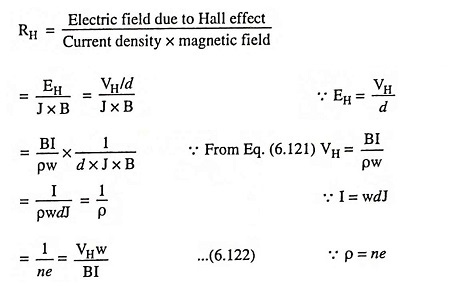In any extrinsic semiconductor, the conductivity is due to charge of one type. Hence the conductivity σ is given as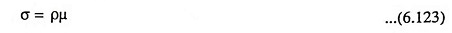If the conductivity σ is measured together with the Hall coefficient, the mobility μ can be determined from the relation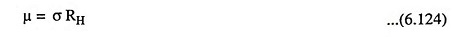The above equation is derived by considering that all the charge carriers move with the mean drift velocity v. Actually the charge carriers have a random thermal distribution in speed. If such distribution is taken into account, the Eq. (6.124) is modified as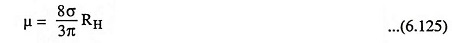### Hall Angle:

The net electric field E in the speci­men is a vector sum of electric field component in the X-direction because of flow of current, Ex and electric field due to Hall Effect in Semiconductor i.e., EH, as shown in Fig. 6.35. Fig. 6.35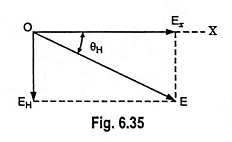So resultant electric field E acts at an angle of θH to the X-axis and this angle is called Hall angle.

Thus, Hall angle,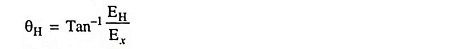From Eq. (6.119),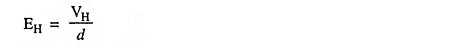and electric field component in the X-direction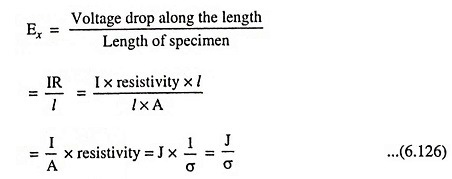Substituting the values of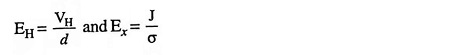in expression for Hall angle we have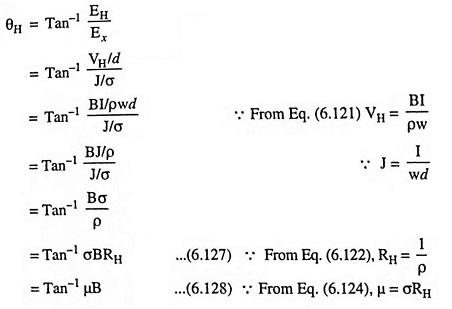### Applications of Hall Effect in Semiconductor:

The Hall Effect in Semiconductor may be used to

• determine whether a semiconductor is of N-type or P-type.
• determine the carrier (electron or hole) concentration.
• measure the conductivity of a material and then compute mobility.
• measure magnetic flux density because Hall voltage VH for a given current, is proportional to magnetic flux density B.
• measure power in an electromagnetic wave.
• measure the displacement.
• measure the current.

The Hall effect multiplier gives the output that is proportional to the product of two signals. If current I is proportional to one input signal and B is linearly related to the other signal then Hall voltage, VH, which is proportional to the product of B and I, is proportional to the product of both signals.

Scroll to Top